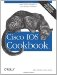# Converting Ethernet and Token Ring MAC Addresses

Problem

You want to convert the bit ordering of MAC addresses to see how they will look after passing through an Ethernet-to-Token Ring bridge.

Solution

The Perl script in Example 15-1 converts Ethernet addresses to the way they will appear when connected through a bridge to a Token Ring. It also performs the reverse translation of Token Ring addresses to Ethernet, which is identical.

Example 15-1. eth-tok-mac.pl

 ```#!/usr/local/bin/perl # # eth-tok-mac.pl -- a script to convert Ethernet to Token Ring MAC # addresses when bridging with RSRB or DLSw # \$convert = "0"; \$convert = "8"; \$convert = "4"; \$convert = "C"; \$convert = "2"; \$convert = "A"; \$convert = "6"; \$convert = "E"; \$convert = "1"; \$convert = "9"; \$convert = "5"; \$convert = "D"; \$convert = "3"; \$convert = "B"; \$convert = "7"; \$convert = "F"; if(\$#ARGV != 0) {usage( );} \$input_MAC = \$ARGV; # first split the incoming MAC into bytes \$_ = \$input_MAC; s/[.:-]//g; for (\$i=0; \$i*2 < length(\$_); \$i++) { @input_bytes[\$i] = substr(\$_, \$i*2, 2); } for (\$i=0; \$i <= \$#input_bytes; \$i++) { \$_ = @input_bytes[\$i]; # first check that there aren't any illegal characters in this address if(/[^0-9a-fA-F]/) { usage( ); } if (length( ) == 2 ) { @output_bytes[\$i] = \$convert[hex(substr(\$_, 1, 1))] . \$convert[hex(substr(\$_, 0, 1))]; } else { usage( ); } } print "the resulting MAC is: "; for (\$i=0; \$i < \$#input_bytes; \$i++) { print "@output_bytes[\$i]-"; } print "@output_bytes[\$#input_bytes] "; sub usage( ) { print "usage: eth-tok-mac.pl "; print " where is in the form HH:HH:HH:HH:HH:HH "; print " or HH-HH-HH-HH-HH-HH or HHHH.HHHH.HHHH print " (H is a hex number 0-F) "; print "The output is the converted MAC address. "; print "Note that this conversion is exactly the same whether converting "; print "from Ethernet to Token Ring or Token Ring to Ethernet. "; exit; }```

The program is run as follows:

```\$ eth-tok-mac.pl 00-00-0c-f0-84-60
the resulting MAC is: 00-00-30-0f-21-06```

Discussion

Token Ring uses a convention of most significant bit first when writing a byte. Ethernet, on the other hand, puts the least significant bit first. So when a bridge connects these two media, the MAC addresses of devices on the Ethernet side will look unfamiliar when viewed from the Token Ring side, and vice versa. The rule for converting from one to the other is relatively simple, however, because it just reflects this reversing of the bit ordering.

Table 15-3 shows how the conversion algorithm works.

Table 15-3. Converting Token Ring to Ethernet MAC addresses

Token Ring Ethernet
Hex Decimal Binary Binary Decimal Hex
0 0 0000 0000 0 0
1 1 0001 1000 8 8
2 2 0010 0100 4 4
3 3 0011 1100 12 C
4 4 0100 0010 2 2
5 5 0101 1010 10 A
6 6 0110 0110 6 6
7 7 0111 1110 14 E
8 8 1000 0001 1 1
9 9 1001 1001 9 9
A 10 1010 0101 5 5
B 11 1011 1101 13 D
C 12 1100 0011 3 3
D 13 1101 1011 11 B
E 14 1110 0111 7 7
F 15 1111 1111 15 F

This table converts each individual hex character in a MAC address, but there is more to it than this because a byte is eight bits long, not four bits like a hex character.

Suppose the Ethernet address is 0000.0cf0.8460. The third byte of this address is 0c. To figure out how this byte will look on the Token Ring side of the bridge, you first switch the two hex characters to get c0. Then convert each of these characters by using Table 15-1. The c becomes 3 and the 0 stays the same, giving a final value of 30. So converting the entire address similarly gives 00-00-30-0f-21-06.

The script provides an automated way to do these translations. If the 8 bits in a byte are numbered from 18, this algorithm simply flips the order so that they appear from 81. This is clearly identical to the inverse translation where the bit order is converted from 81 to 18. So both the script and the above manual technique work identically when converting Ethernet addresses to Token Ring or Token Ring addresses to Ethernet.

Recipe 15.3Cisco IOS Cookbook (Cookbooks (OReilly))
ISBN: 0596527225
EAN: 2147483647
Year: 2004
Pages: 505